Properties and Solutions of Triangles for IIT JEE

Types, Properties and Solutions of Triangles

In, this chapter we are going to discuss about Triangle properties and also its solutions. We all know that triangle consists of three angles & three sides. It is a closed figure made up of three line segments.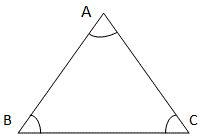In the figure above, the three line segments are AB, BC, CA and the three angles are denoted as ∠​A, ∠​B, ∠​C.

The triangle can be classified into three diffetrent types based on the sides. These are as follows:

• 1) Isosceles triangle

• 2) Scalene triangle

• 3) Acute-angled triangle

• 1)​Isosceles triangle:

A triangle that consists of 2 sides of equal length is known as an Isosceles triangle. In this, the two angles opposite to the equal sides are equal.

• 1) ​Scalene triangle:

• ​A Scalene triangle is a kind of a triangle that consists of three sides of different lengths.

• 1) ​Acute-angled triangle:

• A triangle in which all the angles are acute is called an acute-angled triangle. It is also called as Acute triangle.

The triangle can be classified into three types based on angle are as follows:

• 1) Acute-angled triangle

• 2) Obtuse-angled triangle

• 3) Right-angled triangle

• 1) Acute-angled triangle:

• A triangle in which all the angles are acute is called as an acute-angled triangl

• 2) Obtuse-angled triangle:

• A triangle in which one of the angles is obtuse is called an obtuse-angled triangle.

• 3) Right-angled triangle:

• A triangle in which one of the angles is a right-angle is called as right-angled triangle or right triangle. In the figure below, the side opposite to the right angle, BC is called as the hypotenuse.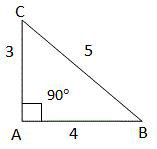For the Right angled triangle ABC, BC​2= AB​2+ AC​2

This theorem is called as ​Pythagorean Theorem.

In the above drawn triangle, 5​2= 3​2+ 4​2.
Those triangle which satisfy this specific condition are known as right angle triangles.

So, the Pythagorean Theorem helps to find whether a triangle is Right-angled (or) not

There are different types of right angles. As of now, our focus is only on a special pair of right angles they are,

• 1. Triangle 45-45-90

• 2. Triangle 30-60-90

• Triangle 45-45-90:

As the name indicates, a 45-45-90 triangle, is the right triangle in which the other 2 angles are 45° each.

It is an isosceles right angle triangle.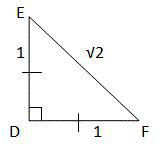In this figure we can view that the angle ∆ DEF, DE = DF and finally ∠​D = 90°.

The sides values are 45-90-45 and the triangle is in the ratio 1: √2 : 1

Triangle 30-60-90:

A 30-60-90 triangle, as the name indicates, it is the right triangle in which the other two angles are 30° and 60°.

It is a scalene right triangle in this none of the sides or the angles are equal.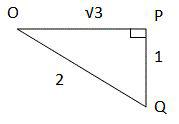The sides in a 30-60-90 triangle are in the ratio 1 : √3 : 2

Like other right triangle, these two angles satisfy the Pythagorean Theorem.

Now, let us discuss about the properties of triangle in details:

• • The first property is angle-sum property. In this, the sum of the angles in a triangle is 180°.

• • The total sum of the lengths (L) of any two sides of a triangle is greater than the length of the third side. Like the difference between the lengths of any two sides of a triangle is less than the length of the third side.

• • The side opposite to the largest angle is the longest side of the triangle & the side opposite to the smallest angle is the shortest side of the triangle.

•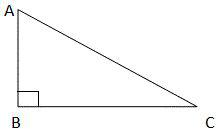In the figure above, the angle ∠​B is the largest angle and the side which is opposite to it, that is, hypotenuse, is the largest side of the triangle.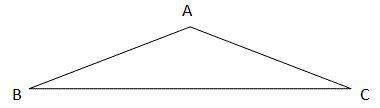In the figure above, the angle ∠​A is the largest angle and the side opposite to it, the portion BC is the largest side of the triangle.

• • Exterior angle property of a triangle: It is an exterior angle of a triangle which is equal to the sum of its interior opposite angles.

•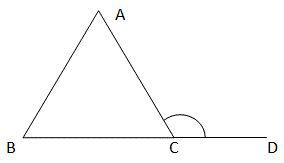Here, the angle ∠​ACD is the exterior angle to the ∆ABC.

Exterior angle property is as ∠​ACD = ∠​CAB + ∠​ABC.

One more important point is:

• 1. The shortest side of a triangle is always found opposite to the smallest interior angle of a triangle.

• 1. And, alternatively one more consequent point is that the longest side is always found to be opposite to the largest interior angle of a triangle.

• Now, we discuss about the Solutions of Triangle:

In typical point of view, a triangle has six main characteristics. It contains of three linear (side lengths ​a, ​b, ​c) and three angular (​α,​ β,​ γ). The classical plane trigonometry problem is to specify three of the six characteristics and to determine the other three.

The triangle can be specifically determined in this method when given any of the following:

• • Three sides (​SSS​)

• • A side, the angle adjacent to it and an angle also opposite to it (​AAS​).

• • 2 sides of a triangle are included and an angle not included between them (​SSA​), if the side length adjacent to the angle is lesser than the other side length.

• • A side and the 2 angles are found adjacent to it (​ASA​)

• • 2 sides and the included of angles of triangle (​SAS​)

• • Finally, 3 angles (​AAA​) on the sphere (but not in the plane).

• In the plane for all the cases, at least one of its side lengths must be specified. At the time if only the angles are provided, the side lengths will be unable to determined, because any similar ​kind of a triangle is the solution.

In this we are going to discuss about the important 3 characteristics as follows:

• (1) ​Side-Side-Side (SSS) criterion for congruence​:

• If all the three sides of a triangle are equal to the respect to three sides of another triangle, then that triangle angles are said to be congruent.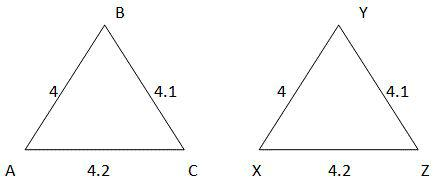Now, ∆ ABC ≅​∆ XYZ as AB = XY, BC = YZ and AC = XZ.

• (1) ​Side-Angle-Side (SAS) criterion for congruence​:

• If only 2 sides and the angle included between the 2 sides of a triangle are equal to the respective 2 sides and the included angle of another triangle, then the triangles are congruent.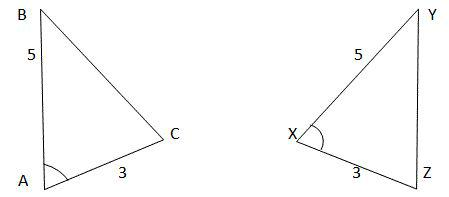Now, ∆ ABC ≅​∆ XYZ as AB = XY, ∠​A = ∠​X and AC = XZ.

• (1) ​Angle-Side-Angle (ASA) criterion for congruence: If 2 angles and the included side of a triangle are found to be same to the corresponding 2 angles and the considered side of other triangle, this kind of triangle is said to be congruent.

•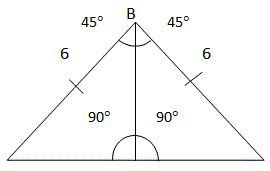We, found in above figure that, ∆ ABD ≅​∆ CBD in which (approx.) the values of ∠​ABD =∠​CBD, the values of AB = CB and the values of∠​ADB =∠​CDB

Let's enter into discussion of triangle area calculation with the following simple formula:

Triangle area calculation:

Triangle is also known as a polygon with the three edges and the three vertices. A triangle is one of the basic shapes of geometry.

Area of a triangle can be calculated by simple formula mentioned below:
The Area of a triangle = (1/2) *Value of Base *Value of Height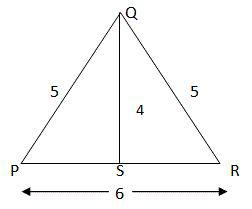To find the area of a triangle, we draw a straight line (or) perpendicular line from the base to the opposite vertex which provides the height of the triangle.

Hence, the area of the ∆ PQR = (1/2) * (PR * QS) = (1/2) * 6 *4 =12 sq. units.

For a right angled triangle, it is easy to determine the area as there is a side perpendicular to the base of the triangle, so we can consider it as a height.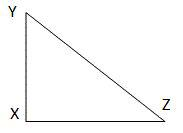The H, which means Height of the ∆ XYZ is XY and its area is (1/2) * XZ * XY and is measured in Sq.Units. Now, we can discuss about finding the area of an obtuse triangle LMN?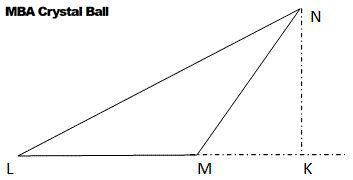In case of an obtuse triangle, when we extend the base and draw a line perpendicular from the vertex to the extended base, it becomes the height of the triangle.

Now, the area of the triangle ∆ LMN = (1/2) * LM(base) * NK(height) sq. units.

It will be clearly known with the help of the following simple example:

If ∆ ABC = 3 (∆ DEF), see the below statements and found the correct one?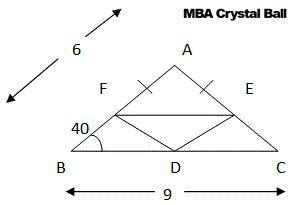A. ∠​E = ∠​F = 120°, ∠​D = 40° AND DE = DF = 3 and EF = 2
B. ∠​E = ∠​F = 110°, ∠​D = 40° AND DE = DF = 3 and EF = 2
C. ∠​F = ∠​E = 40°, ∠​D = 100° AND DF = DE = 2 and EF = 3
D. ∠​E = ∠​F = 110°, ∠​D = 40° AND DE = DF = 3 and EF = 3

Solution: C

Definition for perimeter:

A triangle perimeter can be defined as a total distance around outside of the triangle which can be determined by adding together the length of each side as per the formula:

Perimeter = a+b+c